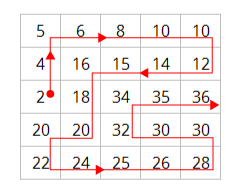# November 2017 Puzzle Periodical - Twisted Sequences

By Wendell W., NSA Mathematician

# November 2017 Puzzle Periodical - Twisted Sequences

## Can you find the missing whole numbers?

### Problem:

Today's NSA Puzzle Periodical challenges you with twisted sequences. This is similar to other number puzzles that require players to fill the blocks of a grid (a square or other shape) with a set range of whole numbers according to a set of rules.

The twisted sequence grid is partially filled with whole numbers. The player's task is to fill the blanks in the grid with the missing whole numbers to complete a sequence. There is only one way to complete the grid correctly.

### The Rules:

In Twisted Sequences, two familiar non-decreasing sequences of positive integers are interleaved to form a single non-decreasing sequence. (A non-decreasing sequence is one in which no element is larger than the one that follows it.) For instance, we could interleave the even numbers (2, 4, 6, 8, 10, …) with the multiples of 5 (5, 10, 15, 20, …) to form the new non-decreasing sequence (2, 4, 5, 6, 8, 10, 10, 12, 14, 15, 16, 18, 20, 20, …). The resulting sequence is then twisted into the shape of a square so each entry is horizontally or vertically adjacent to the number that follows it in the sequence. Here is an example square:

 5 6 8 10 10 4 16 15 14 12 2 18 34 35 36 20 20 32 30 30 22 24 25 26 28Rules Square 1 (Illustration of chart): A curving red line drawn through Rules Square 1 illustrates the twisted number sequence beginning with 2 and then stretching above to 4. The red line then crosses horizontally to the right across the top row of: 5, 6, 8, 10, and 10 and then down to line 2 to: 12, 14, 15, and 16 and then down to 13. The red line then extends down again to 20 and over to the left to 20 and down to 22, then the red line crosses the bottom row to the right to 24, 25, 26, and 28 and then up to 30 and over to the left to 30, then to 32, and up to 34, 35, and 36.

To make things more challenging, certain entries are deleted from the square, as has been done here:

 ? 6 8 ? 10 4 16 15 14 12 2 18 ? 35 36 ? 20 ? ? 30 22 24 25 26 28

Your job is to recover the missing entries.

In this month's Twisted Sequences Puzzle, the first 18 elements of two distinct, non-decreasing sequences of positive integers have been twisted together. Please recover the entire square.

 ? ? ? 19 ? ? 3 3 1 17 ? 29 5 ? 13 ? 34 ? ? 8 11 ? 59 41 ? 610 ? ? ? 43 1597 ? ? 144 53 ?Solution Square (Illustration of chart): A curving red line drawn through the Solution Square illustrates the twisted number sequence beginning in the middle of the row 2 on number 1 and extends up to 1 and then to the left to 2, and then further left to 2, and then down to 3 and over to the right to 3, then down to 5 and over to the left to 5 and then down to 7 and across to the right to 8 and 11 in row 4. The red line then extends directly above to 13 and over to the right to 13. The line then continues straight up to 17 and 19. The red line then moves to the right to 21 and 23 and then straight down to 29. The red line then moves left to 31, down to 34, and to the right to 37, then straight down to 41, 43, and 47. The red line continues left on the bottom row to 53 and then straight up to 55 and 59, then to the left to 61 and then straight down to 89 and 144 on the bottom row. The red line then moves left to 233, then directly above to 377 and then to the left to 610, then down to 987 and then left to 1597 and ends above this at 2584 on line 2.